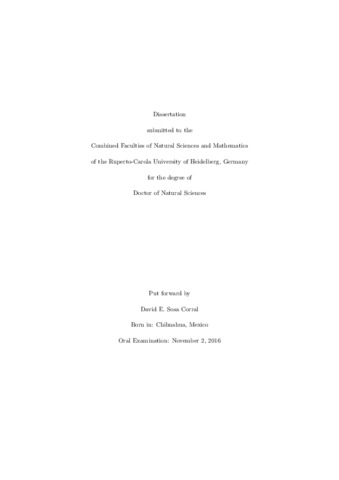## Search for New Physics in the Fully Hadronic \ttbar Channel at $\sqrt{s}= 13$ TeV with the ATLAS Detector at the LHC

Sosa Corral, David E.Preview
PDF, English
A search for heavy resonances where two high transverse momentum top quarks decay hadronically and produce two large jets is carried out using data collected with the ATLAS Detector at the Large Hadron Collider during 2015 at $\sqrt{s}=13 \TeV$. The HEPTopTagger algorithm is used to reconstruct the \ttbar invariant mass and to reduce the large multijet background. The large jets must contain exactly one \bs-tagged jet. The \bs-tagging performance is studied with track and calorimeter jets. The use of track jets for \bs-tagging increases the \ttbar invariant mass tagging efficiency by a factor between 1.3 and 2 and $S/\sqrt{B}$ by 40\%. After the HEPTopTagger application, large jets where a top quark candidate is found, are cleaned from underlying event and pile-up activity and used for the \ttbar invariant mass reconstruction. Using the cleaned large jets instead of the top quark candidate improves the \ttbar invariant mass resolution. The multijet production is estimated using control regions in data. The \ttbar invariant mass distribution is compared for data and Monte Carlo simulation and no significant deviations from the Standard Model predictions are found. Upper limits are set on the production cross section times branching fractions of $Z'$ bosons resonances with masses between 0.75$\TeV$ and 1.65$\TeV$ at 95\% C.L. Expected upper limits using an integrated luminosity of 11.5$\invfb$ collected during 2016 are set on the production cross section times branching fractions of $Z'$ boson with masses between 0.83$\TeV$ and 2.47$\TeV$ at 95\% C.L.